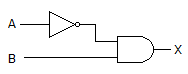# Digital Electronics - Logic Gates

### Exercise :: Logic Gates - Filling the Blanks

6.

A 2-input gate that can be used to pass a digital waveform unchanged at certain times and inverted at other times is a(n) ________.

 A. AND gate B. OR gate C. NAND gate D. XOR gate

Explanation:

No answer description available for this question. Let us discuss.

7.

The gates in this figure are implemented using TTL logic. If the output of the inverter has an internal open circuit, what voltage would you expect to measure at the inverter's output?A. Less than 0.4 V B. 1.6 V C. Greater than 2.4 V D. All of the above

Explanation:

No answer description available for this question. Let us discuss.

8.

When does the output of a NAND gate = 1?

 A. Whenever a 0 is present at an input B. Only when all inputs = 0 C. Whenever a 1 is present at an input D. Only when all inputs = 1

Explanation:

No answer description available for this question. Let us discuss.

9.

The number of input combinations for a 4-input gate is ________.

 A. 9 B. 8 C. 15 D. 16

Explanation:

No answer description available for this question. Let us discuss.

10.

When does the output of a NOR gate = 0?

 A. Whenever a 0 is present at an input B. Only when all inputs = 0 C. Whenever a 1 is present at an input D. Only when all inputs = 1